Скачать презентацию ECON 1001 AB Introduction to Economics I Dr

82baaccd9de9dd0bb445e69c748801df.ppt

• Количество слайдов: 36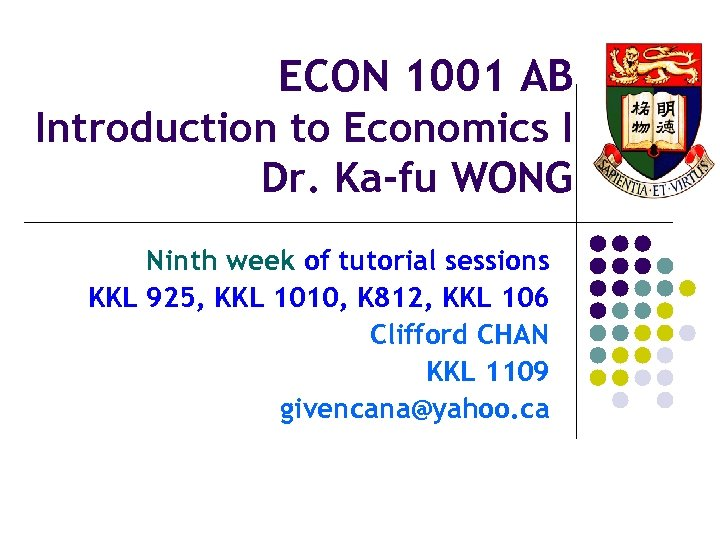ECON 1001 AB Introduction to Economics I Dr. Ka-fu WONG Ninth week of tutorial sessions KKL 925, KKL 1010, K 812, KKL 106 Clifford CHAN KKL 1109 [email protected] ca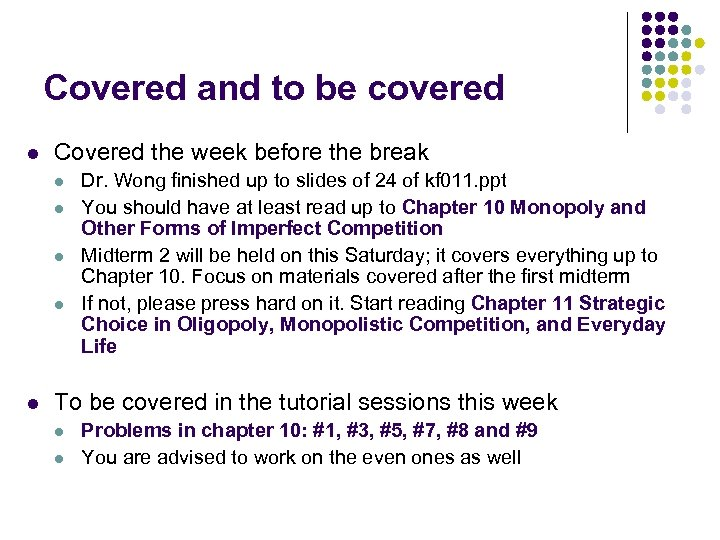Covered and to be covered l Covered the week before the break l l l Dr. Wong finished up to slides of 24 of kf 011. ppt You should have at least read up to Chapter 10 Monopoly and Other Forms of Imperfect Competition Midterm 2 will be held on this Saturday; it covers everything up to Chapter 10. Focus on materials covered after the first midterm If not, please press hard on it. Start reading Chapter 11 Strategic Choice in Oligopoly, Monopolistic Competition, and Everyday Life To be covered in the tutorial sessions this week l l Problems in chapter 10: #1, #3, #5, #7, #8 and #9 You are advised to work on the even ones as well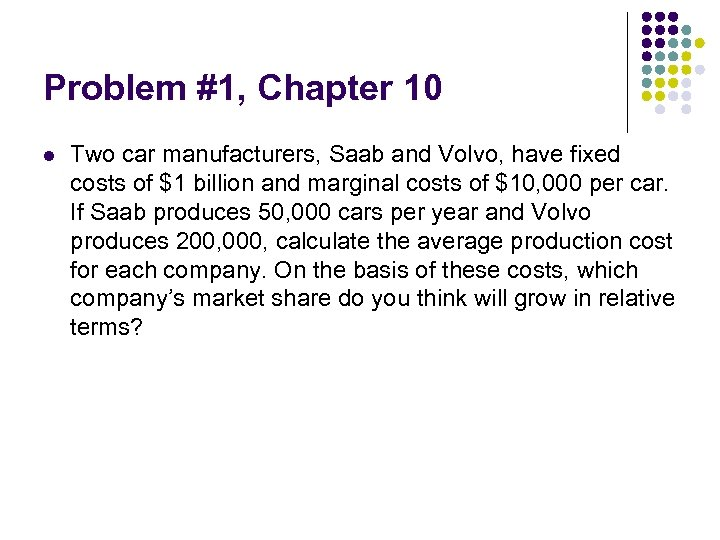Problem #1, Chapter 10 l Two car manufacturers, Saab and Volvo, have fixed costs of \$1 billion and marginal costs of \$10, 000 per car. If Saab produces 50, 000 cars per year and Volvo produces 200, 000, calculate the average production cost for each company. On the basis of these costs, which company’s market share do you think will grow in relative terms?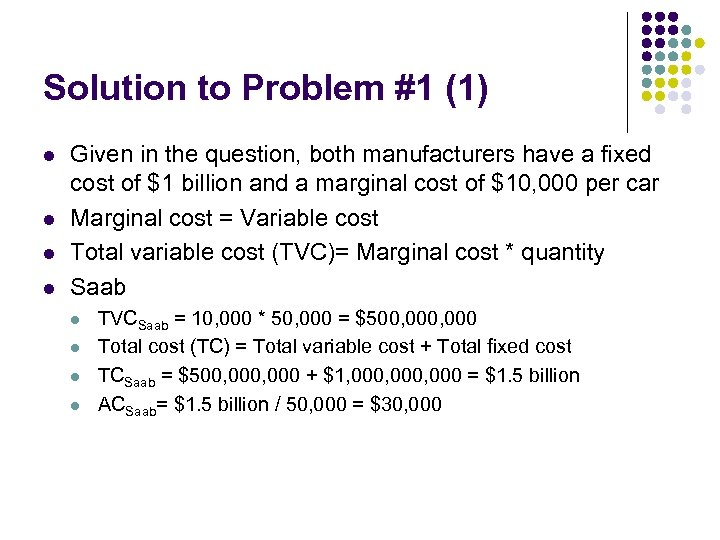Solution to Problem #1 (1) l l Given in the question, both manufacturers have a fixed cost of \$1 billion and a marginal cost of \$10, 000 per car Marginal cost = Variable cost Total variable cost (TVC)= Marginal cost * quantity Saab l l TVCSaab = 10, 000 * 50, 000 = \$500, 000 Total cost (TC) = Total variable cost + Total fixed cost TCSaab = \$500, 000 + \$1, 000, 000 = \$1. 5 billion ACSaab= \$1. 5 billion / 50, 000 = \$30, 000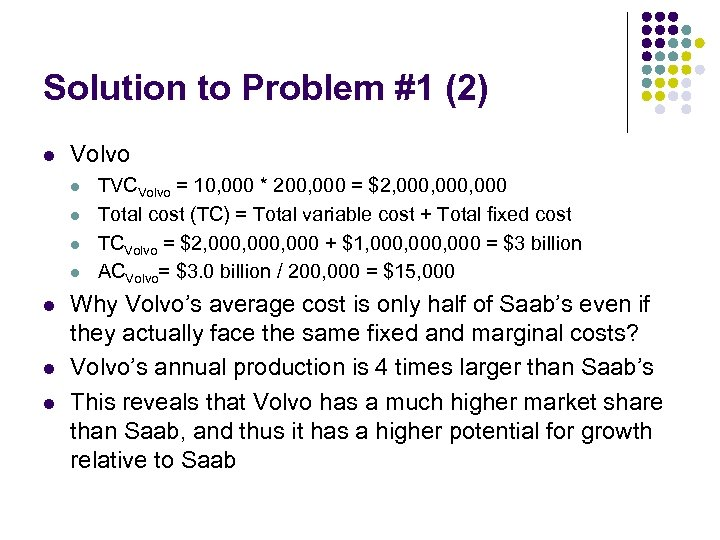Solution to Problem #1 (2) l Volvo l l l l TVCVolvo = 10, 000 * 200, 000 = \$2, 000, 000 Total cost (TC) = Total variable cost + Total fixed cost TCVolvo = \$2, 000, 000 + \$1, 000, 000 = \$3 billion ACVolvo= \$3. 0 billion / 200, 000 = \$15, 000 Why Volvo’s average cost is only half of Saab’s even if they actually face the same fixed and marginal costs? Volvo’s annual production is 4 times larger than Saab’s This reveals that Volvo has a much higher market share than Saab, and thus it has a higher potential for growth relative to Saab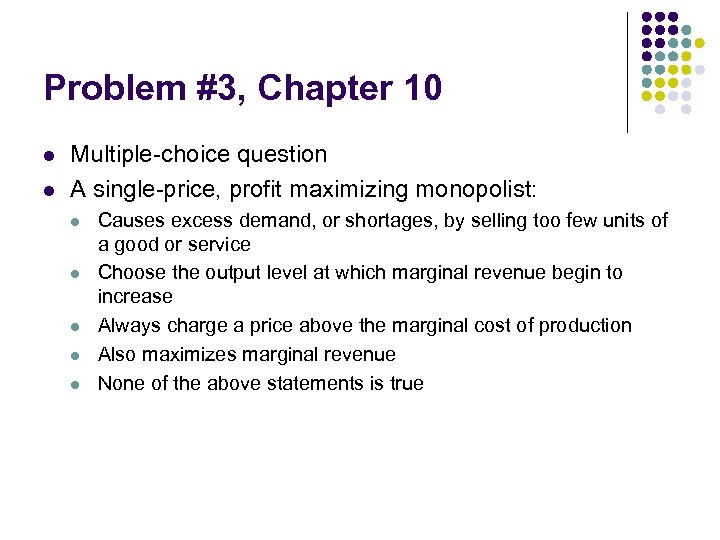Problem #3, Chapter 10 l l Multiple-choice question A single-price, profit maximizing monopolist: l l l Causes excess demand, or shortages, by selling too few units of a good or service Choose the output level at which marginal revenue begin to increase Always charge a price above the marginal cost of production Also maximizes marginal revenue None of the above statements is true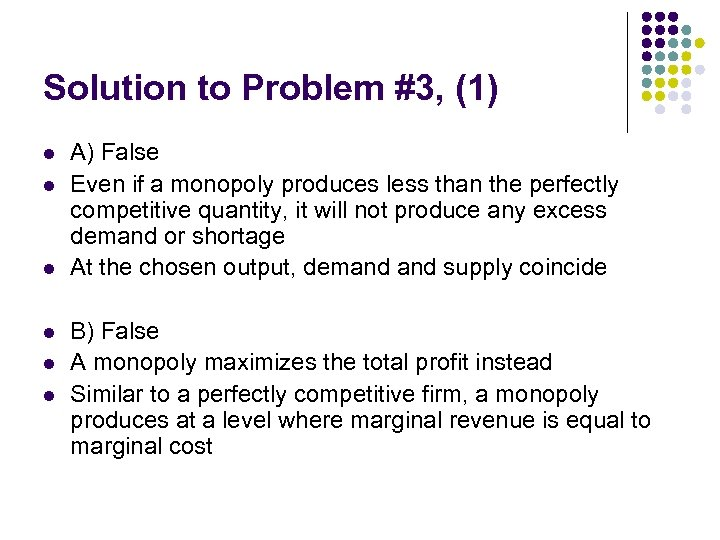Solution to Problem #3, (1) l l l A) False Even if a monopoly produces less than the perfectly competitive quantity, it will not produce any excess demand or shortage At the chosen output, demand supply coincide B) False A monopoly maximizes the total profit instead Similar to a perfectly competitive firm, a monopoly produces at a level where marginal revenue is equal to marginal cost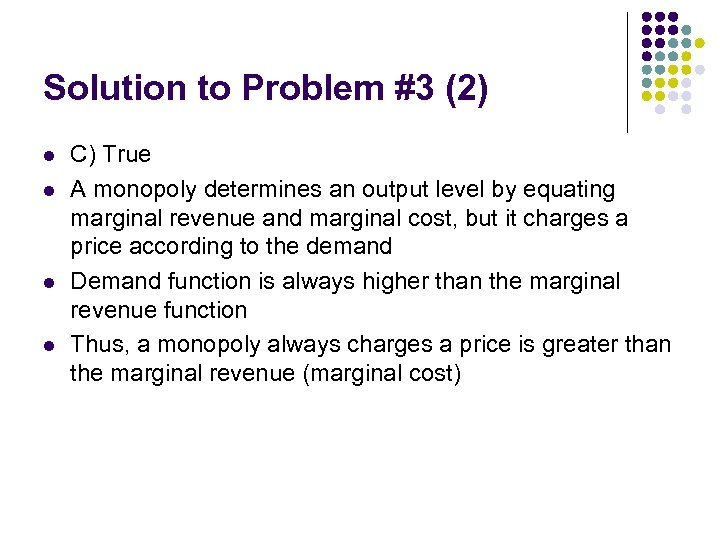Solution to Problem #3 (2) l l C) True A monopoly determines an output level by equating marginal revenue and marginal cost, but it charges a price according to the demand Demand function is always higher than the marginal revenue function Thus, a monopoly always charges a price is greater than the marginal revenue (marginal cost)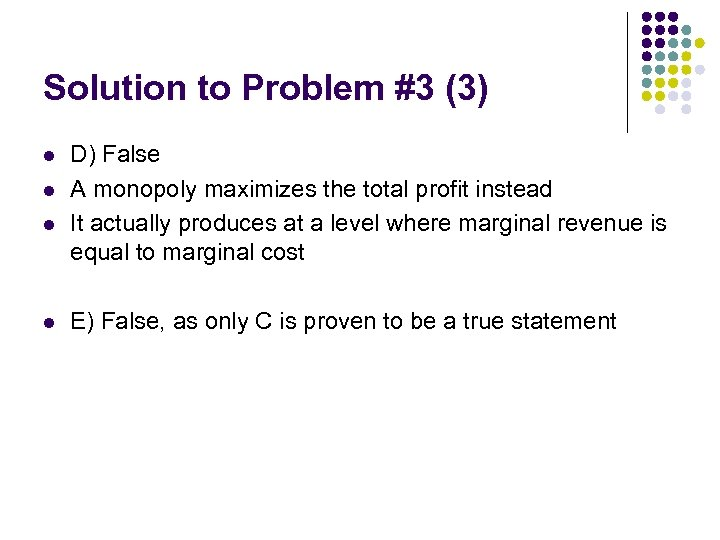Solution to Problem #3 (3) l l D) False A monopoly maximizes the total profit instead It actually produces at a level where marginal revenue is equal to marginal cost E) False, as only C is proven to be a true statement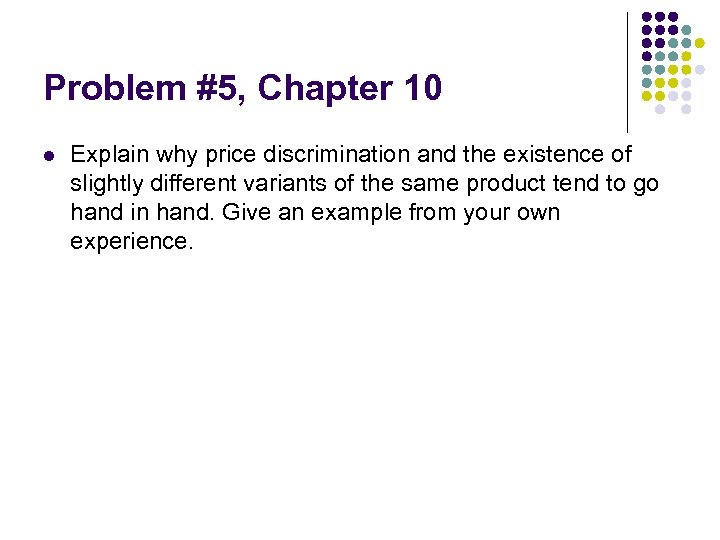Problem #5, Chapter 10 l Explain why price discrimination and the existence of slightly different variants of the same product tend to go hand in hand. Give an example from your own experience.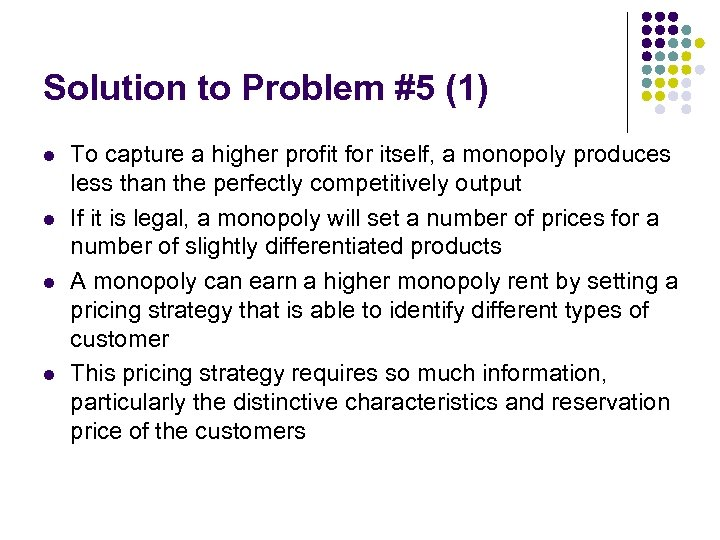Solution to Problem #5 (1) l l To capture a higher profit for itself, a monopoly produces less than the perfectly competitively output If it is legal, a monopoly will set a number of prices for a number of slightly differentiated products A monopoly can earn a higher monopoly rent by setting a pricing strategy that is able to identify different types of customer This pricing strategy requires so much information, particularly the distinctive characteristics and reservation price of the customers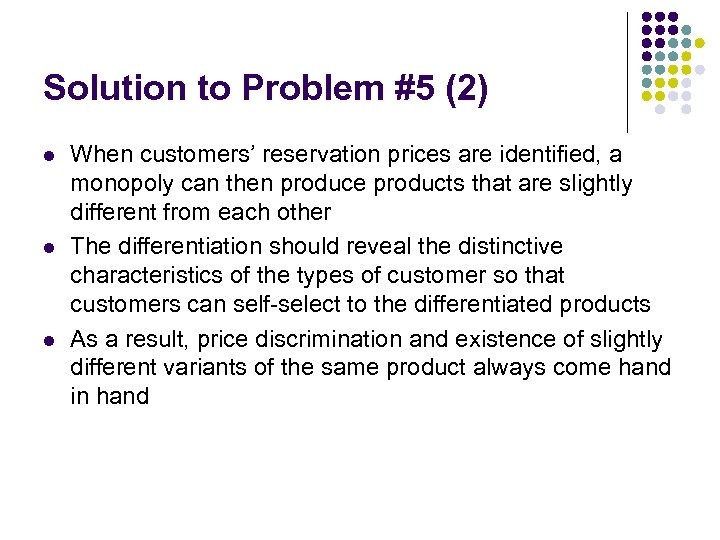Solution to Problem #5 (2) l l l When customers’ reservation prices are identified, a monopoly can then produce products that are slightly different from each other The differentiation should reveal the distinctive characteristics of the types of customer so that customers can self-select to the differentiated products As a result, price discrimination and existence of slightly different variants of the same product always come hand in hand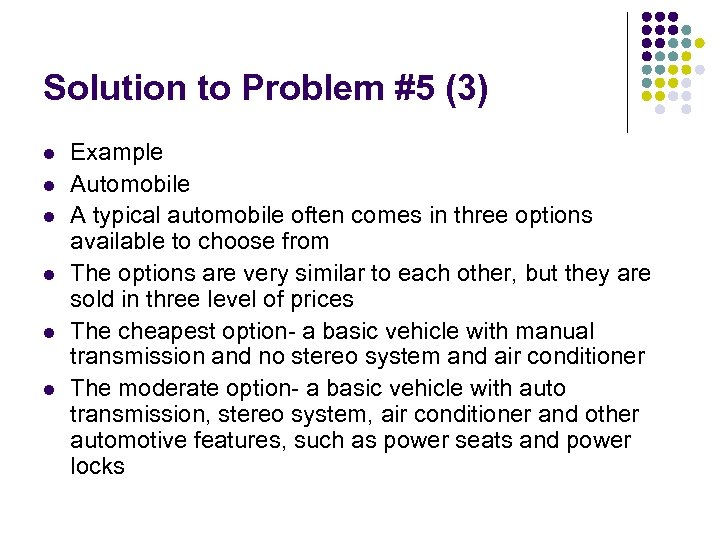Solution to Problem #5 (3) l l l Example Automobile A typical automobile often comes in three options available to choose from The options are very similar to each other, but they are sold in three level of prices The cheapest option- a basic vehicle with manual transmission and no stereo system and air conditioner The moderate option- a basic vehicle with auto transmission, stereo system, air conditioner and other automotive features, such as power seats and power locks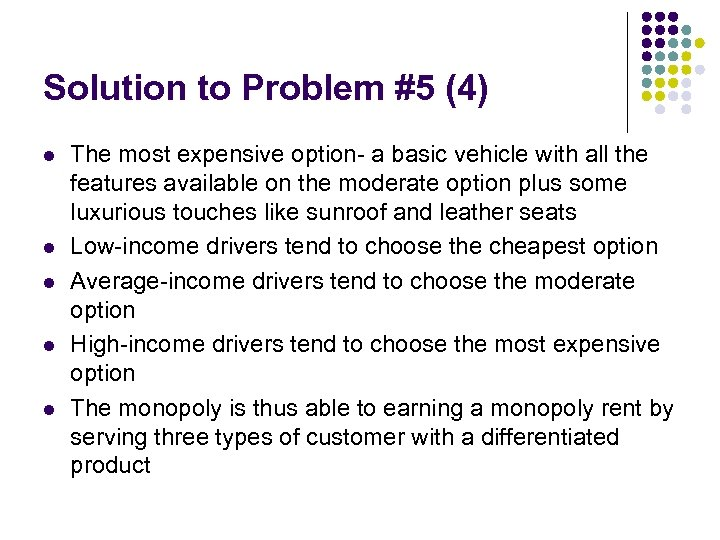Solution to Problem #5 (4) l l l The most expensive option- a basic vehicle with all the features available on the moderate option plus some luxurious touches like sunroof and leather seats Low-income drivers tend to choose the cheapest option Average-income drivers tend to choose the moderate option High-income drivers tend to choose the most expensive option The monopoly is thus able to earning a monopoly rent by serving three types of customer with a differentiated product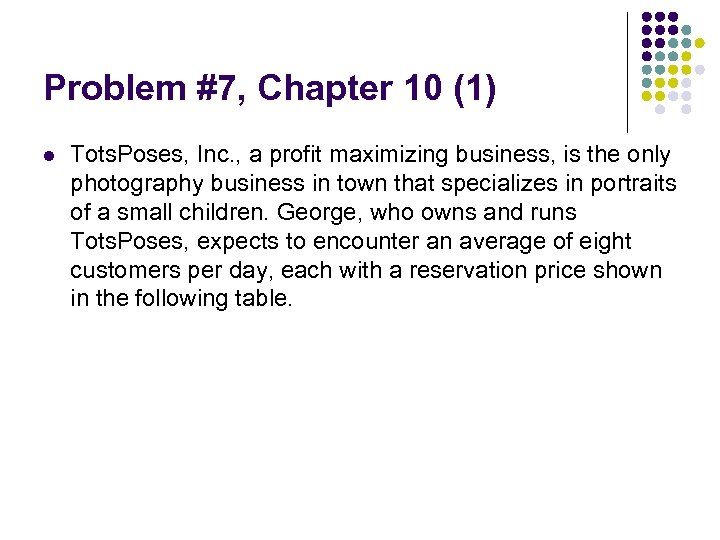Problem #7, Chapter 10 (1) l Tots. Poses, Inc. , a profit maximizing business, is the only photography business in town that specializes in portraits of a small children. George, who owns and runs Tots. Poses, expects to encounter an average of eight customers per day, each with a reservation price shown in the following table.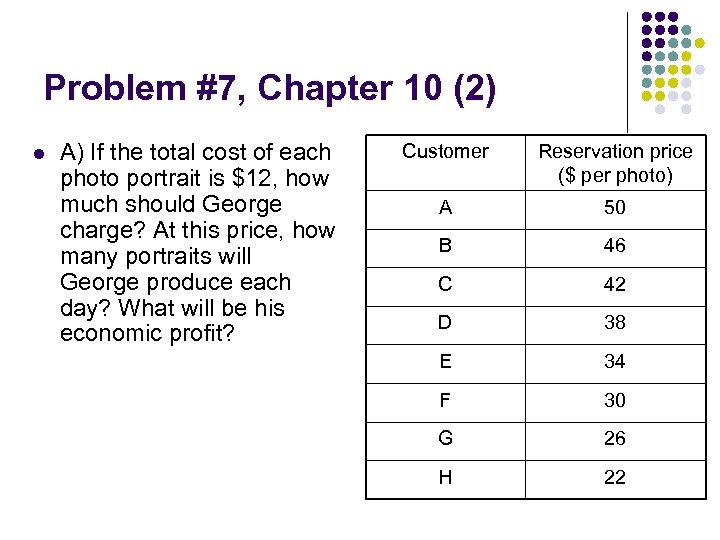Problem #7, Chapter 10 (2) l A) If the total cost of each photo portrait is \$12, how much should George charge? At this price, how many portraits will George produce each day? What will be his economic profit? Customer Reservation price (\$ per photo) A 50 B 46 C 42 D 38 E 34 F 30 G 26 H 22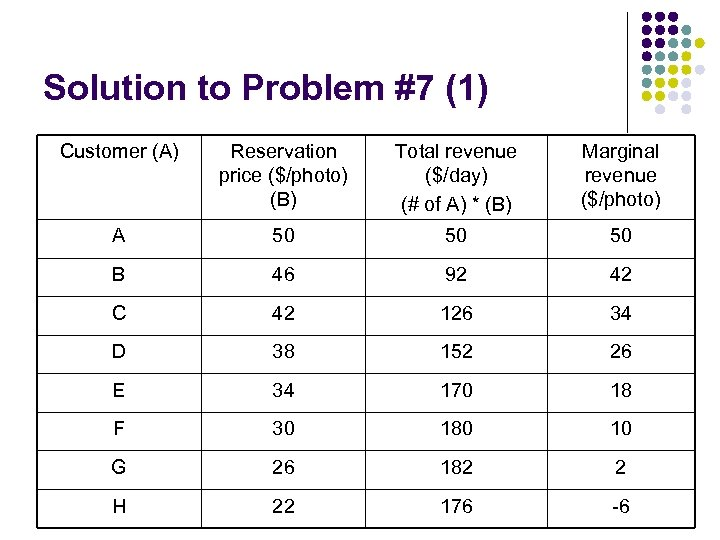Solution to Problem #7 (1) Customer (A) Reservation price (\$/photo) (B) Total revenue (\$/day) (# of A) * (B) Marginal revenue (\$/photo) A 50 50 50 B 46 92 42 C 42 126 34 D 38 152 26 E 34 170 18 F 30 180 10 G 26 182 2 H 22 176 -6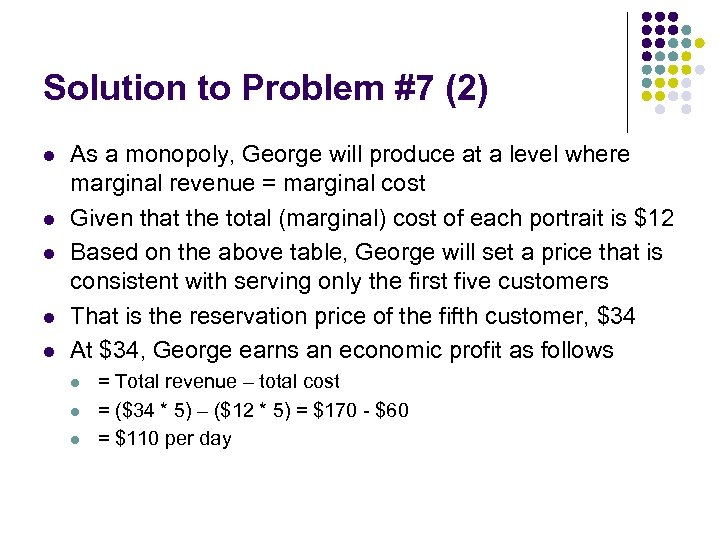Solution to Problem #7 (2) l l l As a monopoly, George will produce at a level where marginal revenue = marginal cost Given that the total (marginal) cost of each portrait is \$12 Based on the above table, George will set a price that is consistent with serving only the first five customers That is the reservation price of the fifth customer, \$34 At \$34, George earns an economic profit as follows l l l = Total revenue – total cost = (\$34 * 5) – (\$12 * 5) = \$170 - \$60 = \$110 per day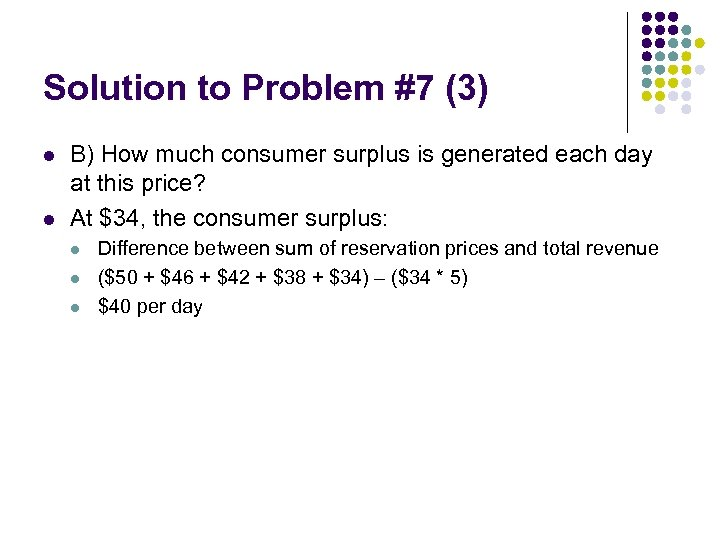Solution to Problem #7 (3) l l B) How much consumer surplus is generated each day at this price? At \$34, the consumer surplus: l l l Difference between sum of reservation prices and total revenue (\$50 + \$46 + \$42 + \$38 + \$34) – (\$34 * 5) \$40 per day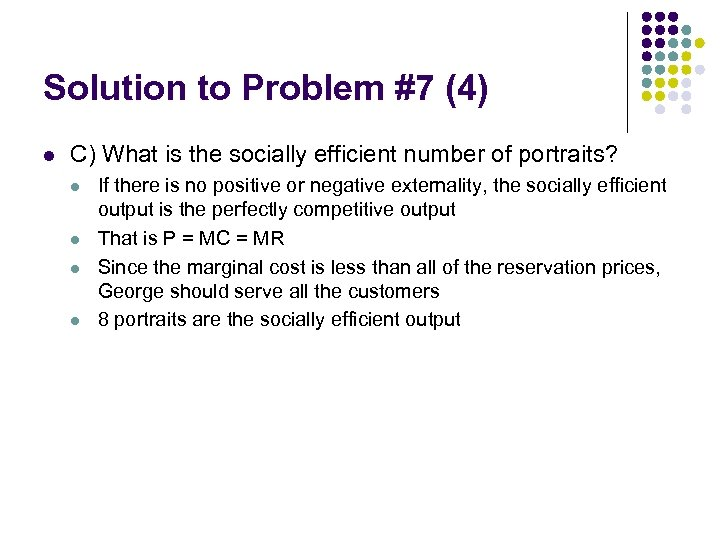Solution to Problem #7 (4) l C) What is the socially efficient number of portraits? l l If there is no positive or negative externality, the socially efficient output is the perfectly competitive output That is P = MC = MR Since the marginal cost is less than all of the reservation prices, George should serve all the customers 8 portraits are the socially efficient output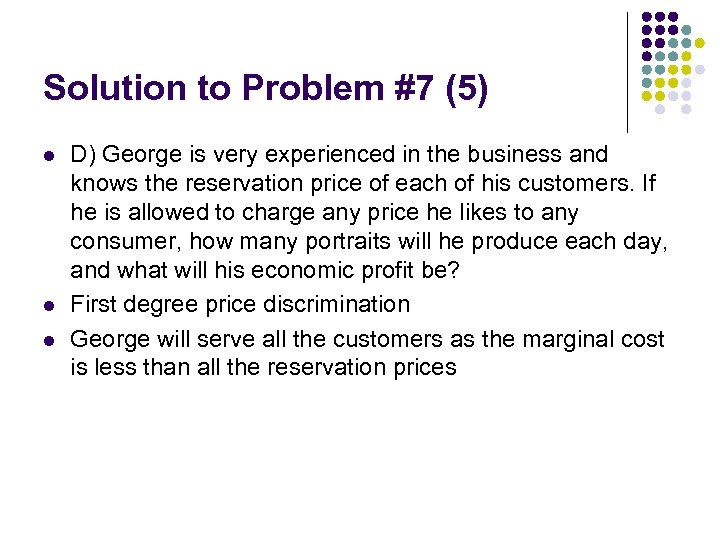Solution to Problem #7 (5) l l l D) George is very experienced in the business and knows the reservation price of each of his customers. If he is allowed to charge any price he likes to any consumer, how many portraits will he produce each day, and what will his economic profit be? First degree price discrimination George will serve all the customers as the marginal cost is less than all the reservation prices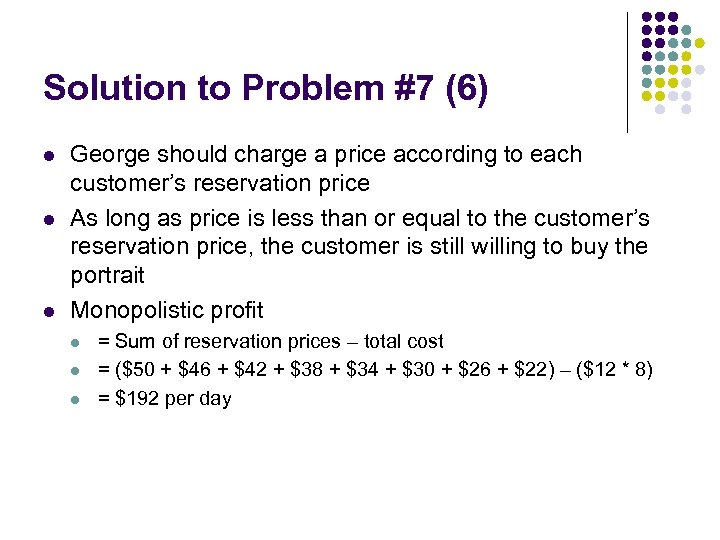Solution to Problem #7 (6) l l l George should charge a price according to each customer’s reservation price As long as price is less than or equal to the customer’s reservation price, the customer is still willing to buy the portrait Monopolistic profit l l l = Sum of reservation prices – total cost = (\$50 + \$46 + \$42 + \$38 + \$34 + \$30 + \$26 + \$22) – (\$12 * 8) = \$192 per day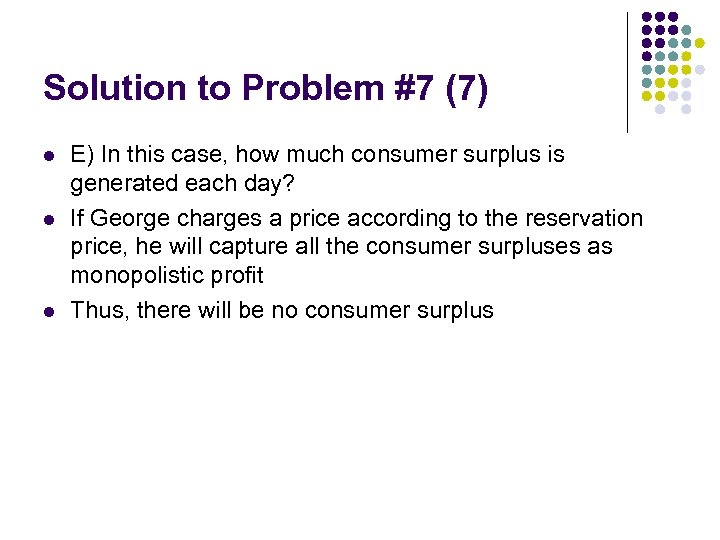Solution to Problem #7 (7) l l l E) In this case, how much consumer surplus is generated each day? If George charges a price according to the reservation price, he will capture all the consumer surpluses as monopolistic profit Thus, there will be no consumer surplus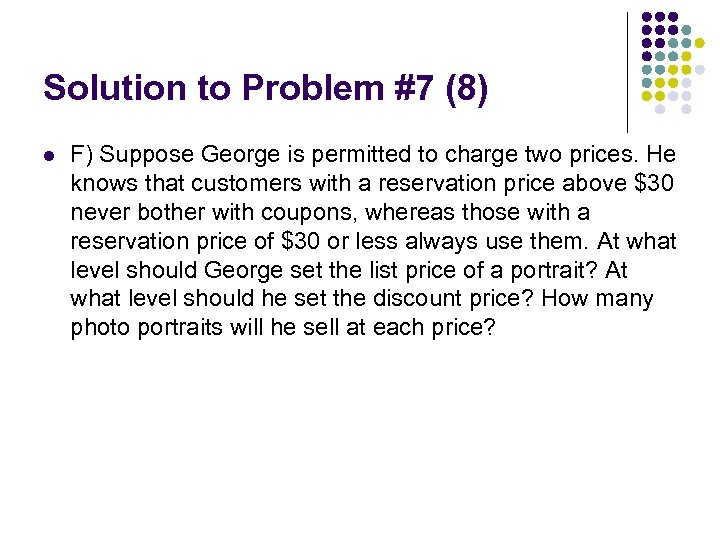Solution to Problem #7 (8) l F) Suppose George is permitted to charge two prices. He knows that customers with a reservation price above \$30 never bother with coupons, whereas those with a reservation price of \$30 or less always use them. At what level should George set the list price of a portrait? At what level should he set the discount price? How many photo portraits will he sell at each price?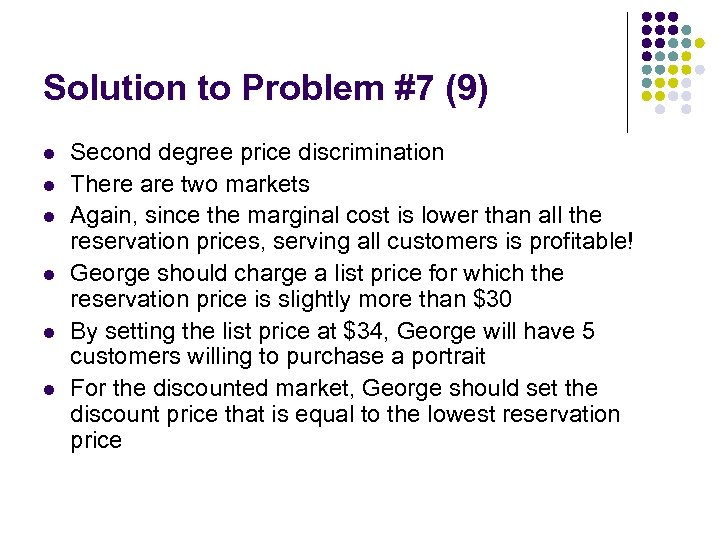Solution to Problem #7 (9) l l l Second degree price discrimination There are two markets Again, since the marginal cost is lower than all the reservation prices, serving all customers is profitable! George should charge a list price for which the reservation price is slightly more than \$30 By setting the list price at \$34, George will have 5 customers willing to purchase a portrait For the discounted market, George should set the discount price that is equal to the lowest reservation price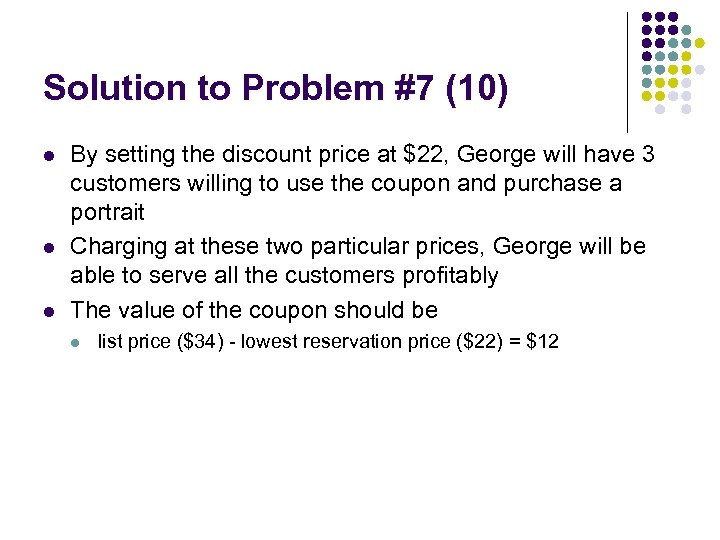Solution to Problem #7 (10) l l l By setting the discount price at \$22, George will have 3 customers willing to use the coupon and purchase a portrait Charging at these two particular prices, George will be able to serve all the customers profitably The value of the coupon should be l list price (\$34) - lowest reservation price (\$22) = \$12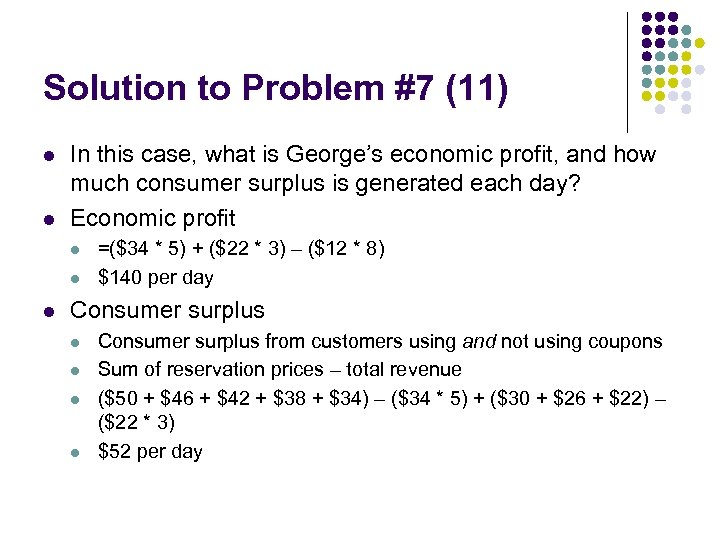Solution to Problem #7 (11) l l In this case, what is George’s economic profit, and how much consumer surplus is generated each day? Economic profit l l l =(\$34 * 5) + (\$22 * 3) – (\$12 * 8) \$140 per day Consumer surplus l l Consumer surplus from customers using and not using coupons Sum of reservation prices – total revenue (\$50 + \$46 + \$42 + \$38 + \$34) – (\$34 * 5) + (\$30 + \$26 + \$22) – (\$22 * 3) \$52 per day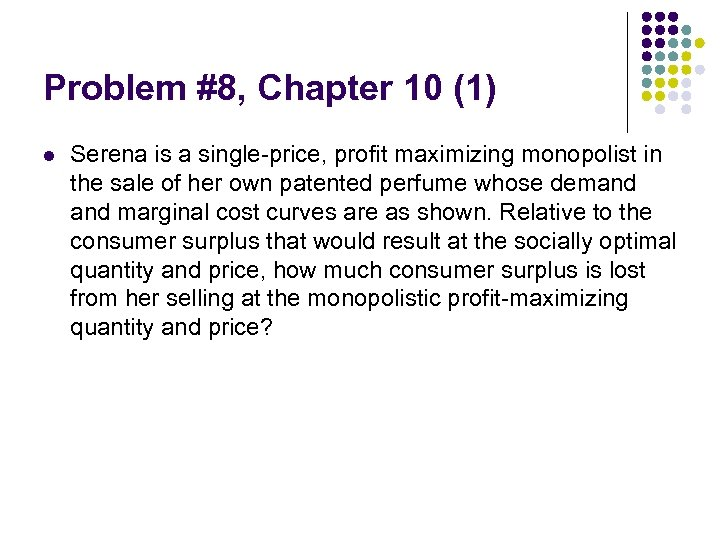Problem #8, Chapter 10 (1) l Serena is a single-price, profit maximizing monopolist in the sale of her own patented perfume whose demand marginal cost curves are as shown. Relative to the consumer surplus that would result at the socially optimal quantity and price, how much consumer surplus is lost from her selling at the monopolistic profit-maximizing quantity and price?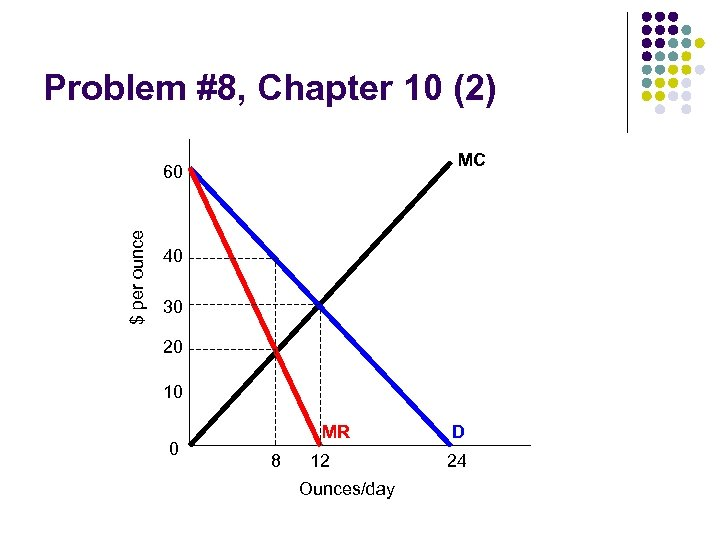Problem #8, Chapter 10 (2) MC \$ per ounce 60 40 30 20 10 0 MR 8 12 Ounces/day D 24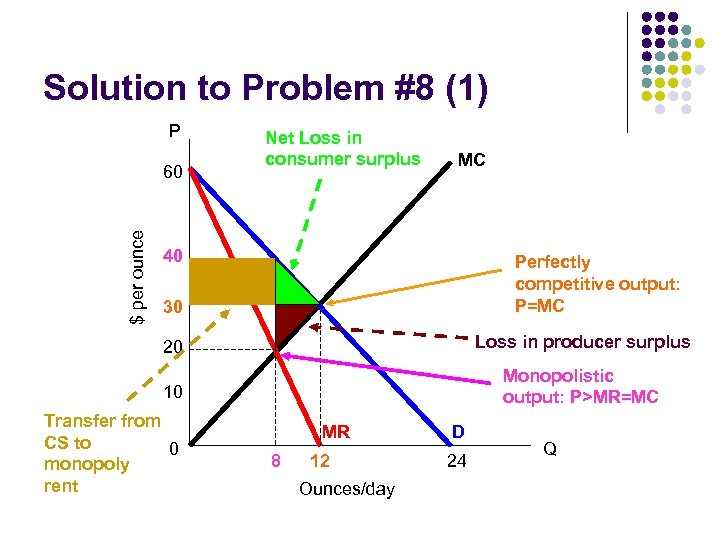Solution to Problem #8 (1) P \$ per ounce 60 Net Loss in consumer surplus MC 40 Perfectly competitive output: P=MC 30 20 Loss in producer surplus 10 Monopolistic output: P>MR=MC Transfer from CS to 0 monopoly rent MR 8 12 Ounces/day D 24 Q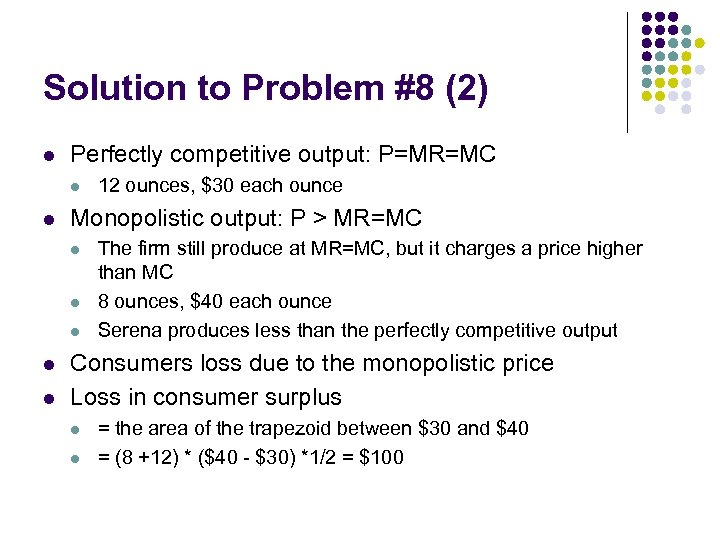Solution to Problem #8 (2) l Perfectly competitive output: P=MR=MC l l Monopolistic output: P > MR=MC l l l 12 ounces, \$30 each ounce The firm still produce at MR=MC, but it charges a price higher than MC 8 ounces, \$40 each ounce Serena produces less than the perfectly competitive output Consumers loss due to the monopolistic price Loss in consumer surplus l l = the area of the trapezoid between \$30 and \$40 = (8 +12) * (\$40 - \$30) *1/2 = \$100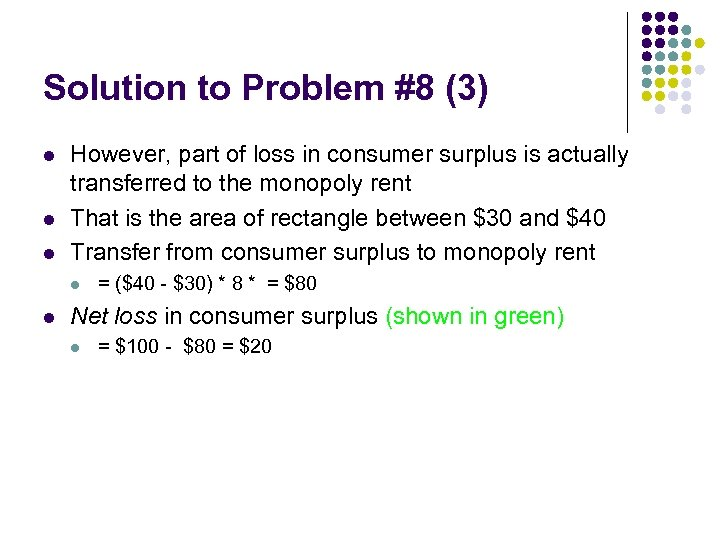Solution to Problem #8 (3) l l l However, part of loss in consumer surplus is actually transferred to the monopoly rent That is the area of rectangle between \$30 and \$40 Transfer from consumer surplus to monopoly rent l l = (\$40 - \$30) * 8 * = \$80 Net loss in consumer surplus (shown in green) l = \$100 - \$80 = \$20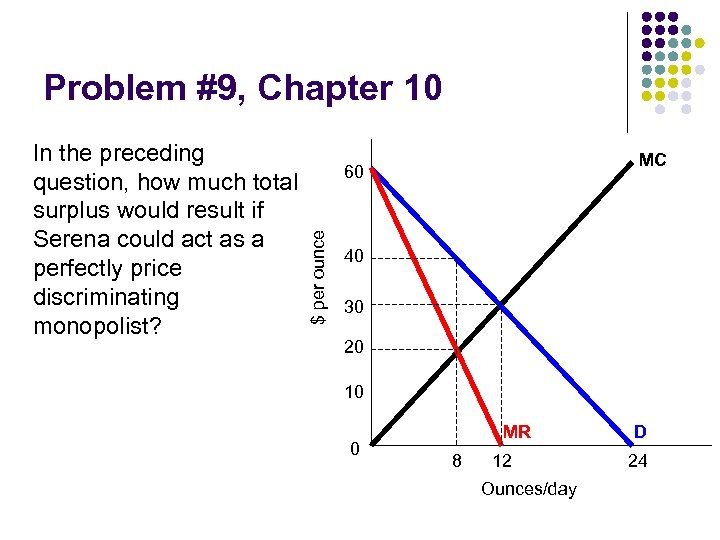Problem #9, Chapter 10 MC 60 \$ per ounce In the preceding question, how much total surplus would result if Serena could act as a perfectly price discriminating monopolist? 40 30 20 10 0 MR 8 12 Ounces/day D 24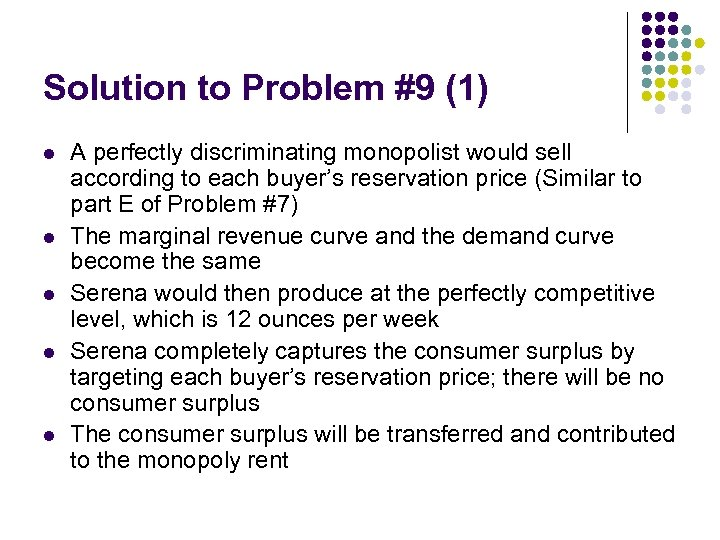Solution to Problem #9 (1) l l l A perfectly discriminating monopolist would sell according to each buyer’s reservation price (Similar to part E of Problem #7) The marginal revenue curve and the demand curve become the same Serena would then produce at the perfectly competitive level, which is 12 ounces per week Serena completely captures the consumer surplus by targeting each buyer’s reservation price; there will be no consumer surplus The consumer surplus will be transferred and contributed to the monopoly rent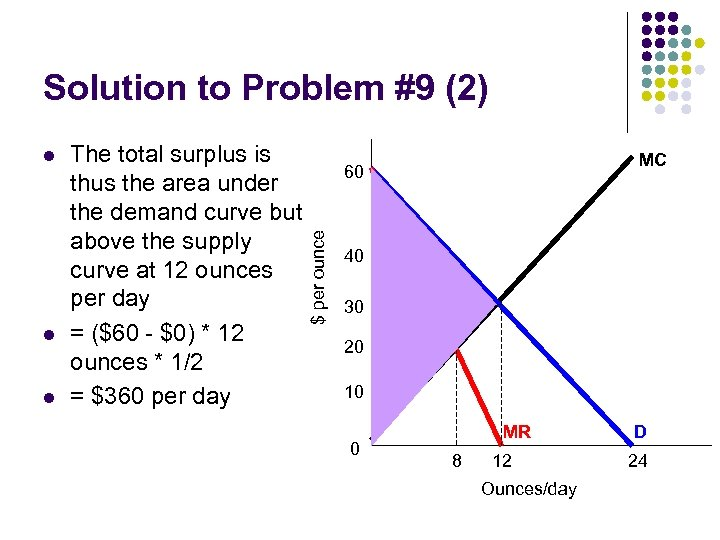Solution to Problem #9 (2) l l The total surplus is thus the area under the demand curve but above the supply curve at 12 ounces per day = (\$60 - \$0) * 12 ounces * 1/2 = \$360 per day MC 60 \$ per ounce l 40 30 20 10 0 MR 8 12 Ounces/day D 24The end Thanks for coming! See you next week!!!